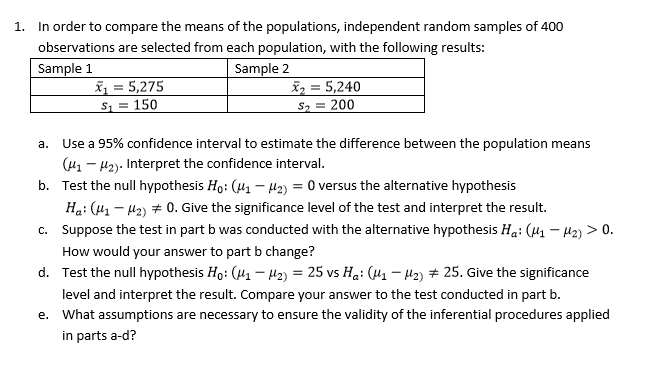# In order to compare the means of the populations, independent random samples of 400 observations are selected from each population, with the following results: 1. Sample 1 Sample 2 5,275 5,240 2 200 S1 150 Use a 95% confidence interval to estimate the difference between the population means a. (u1-12). Interpret the confidence interval. b. Test the null hypothesis Ho: (H1 H2)0 versus the alternative hypothesis Hai (11-12) # 0, Give the significance level of the test and interpret the result. c. suppose the test in part b was conducted with the alternative hypothesis Ha: (μ1-μ2) > 0. How would your answer to part b change? Test the null hypothesis Ho: (4-2,-25 vs Ha: (11-Ha) # 25, Give the significance d. level and interpret the result. Compare your answer to the test conducted in part b. What assumptions are necessary to ensure the validity of the inferential procedures applied e. in parts a-d?

Question

Part A, B, Chelp_outlineImage TranscriptioncloseIn order to compare the means of the populations, independent random samples of 400 observations are selected from each population, with the following results: 1. Sample 1 Sample 2 5,275 5,240 2 200 S1 150 Use a 95% confidence interval to estimate the difference between the population means a. (u1-12). Interpret the confidence interval. b. Test the null hypothesis Ho: (H1 H2)0 versus the alternative hypothesis Hai (11-12) # 0, Give the significance level of the test and interpret the result. c. suppose the test in part b was conducted with the alternative hypothesis Ha: (μ1-μ2) > 0. How would your answer to part b change? Test the null hypothesis Ho: (4-2,-25 vs Ha: (11-Ha) # 25, Give the significance d. level and interpret the result. Compare your answer to the test conducted in part b. What assumptions are necessary to ensure the validity of the inferential procedures applied e. in parts a-d? fullscreen